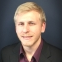# QlikView App Dev

Discussion Board for collaboration related to QlikView App Development.

cancel
Showing results for
Did you mean:Contributor III

## Setting multiple field values in a variable

I am trying to write a previousQuarter variable that is comprised of the following:

```= if
(
GetFieldSelections([Quarter]) = 'Q1',
[Deposit Year] = (GetFieldSelections([Deposit Year]) - 1) and (Quarter = 'Q4'),
[Quarter] = 'Q' & (Right(Quarter, 1) - 1)
)

```

I need this variable to use in pulling the previous quarter balance, and the if() here needs to be able to handle when Q1 is selected and so there needs to be a comparison to the previous year's Q4 balance.

I think that maybe "[...] = x" is not appropriate syntax; so is there a proper way to write this, or am I going about this the wrong way?

Thanks!

Message was edited by: Tyler Tollin

1 Solution

Accepted SolutionsMVP

Try this

=num

(Sum({<[Record Date] = P([Previous Quarter End]), Quarter, Year>}[Current Balance Amount]),

MoneyFormat

)

11 RepliesSpecialist II

may be you can make use of quarterstart and quarterend functions

For current -

Sum({<Date={">=\$(=QuarterStart(max(Date)))<=\$(=QuarterEnd(max(Date)))"}>}YourField)

For previous-

Sum({<Date={">=\$(=QuarterStart(max(Date), -1))<=\$(=QuarterEnd(max(Date), -1))"}>}YourField)MVP

Probably the best way is to Quarter date field in the script from your normal date field: Num(QuarterStart(MyDate))) as YearQuarter. Once you have that field you can create variables for the currently selected and the previous quarter:

vCurrentQuarter: =max(YearQuarter)

vPreviousQuarter: =num(QuarterStart(max(YearQuarter),-1))

You can then use the variables in set analysis expressions:

sum({<YearQuarter={\$(vCurrentQuarter)}>}Amount)

sum({<Year, Quarter, YearQuarter={\$(vPreviousQuarter)}>}Amount)

In the set analysis expression for the previous quarter we add Year, Quarter, to make the expression ignore your selections in the Year and Quarter dimensions so only a selection in the field YearQuarter is applied to this expression so the data is calculated over the correct YearQuarter value, i.e. the previous one with the value in the vPreviousQuarter variable.

talk is cheap, supply exceeds demandContributor III
Author

Thanks for the suggestion, Gysbert. I'm going to try and refactor to get this suggestion to work. If it does, I'll come back and mark as answered.Contributor III
Author

So I haven't had any luck with this, but I've made enough changes to your suggestion to reasonably think that I might have made some mistakes. Here's what I've done so far:

1. I've incorporated the master calendar here:
```MasterCalendar:
TempDate                As [Record Date],
//Week(TempDate)        As Week,
Year(TempDate)          As [Deposit Year],
Month(TempDate)         As [Deposit Month],
//Day(TempDate)         As Day,
'Q' & ceil(month(TempDate) / 3) As [Deposit Quarter],
Date( QuarterStart(TempDate) )  As [Deposit Quarter Start],
MonthStart(Date( QuarterEnd( Date( QuarterStart(TempDate)-1)) )) As [Deposit Previous Quarter End];
//Week(weekstart(TempDate)) & '-' & WeekYear(TempDate) As WeekYear,
//WeekDay(TempDate)     As WeekDay;

//=== Generate a temp table of dates ===
date(mindate + IterNo()) AS TempDate,
maxdate // Used in InYearToDate() above, but not kept
WHILE mindate + IterNo() <= maxdate;

//=== Get min/max dates from Field ===/
min(FieldValue('Record Date', recno()))-1 as mindate,
max(FieldValue('Record Date', recno())) as maxdate
AUTOGENERATE FieldValueCount('Record Date');
```

2. Created the following formula to test:

```=sum
(
{< [Deposit Month], [Deposit Year], [Record Date] = {\$(=\$(previousQtrMonth))} >}
[Current Balance Amount]
)
```

Which uses the following variable:

```previousQtrMonth = [Deposit Previous Quarter End]
```

Still no dice; the balance formula is returning 0. I've tried all kinds of combinations of ' ' versus " ", variables in the set expression or just the field name, etc. I'm out of ideas.Specialist II

Do you mind sharing sample data and the output required..Contributor III
AuthorMVP

Try this

=num

(Sum({<[Record Date] = P([Previous Quarter End]), Quarter, Year>}[Current Balance Amount]),

MoneyFormat

)Contributor III
Author

Nailed it like always, Sunny. Marked as correct.

I can't find anything on Documentation about P(); would you mind explaining what it does and when to use it?MVP